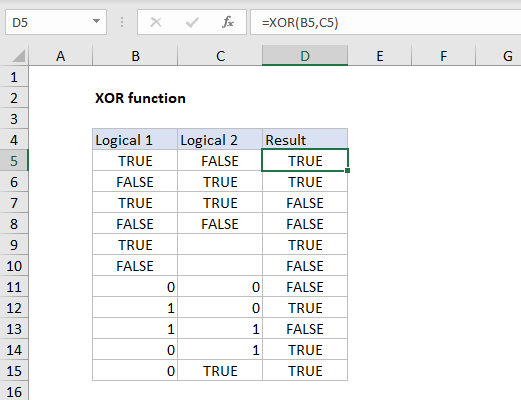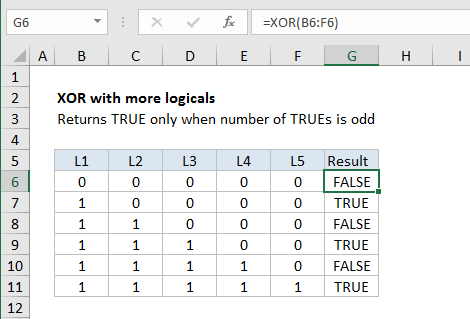Surpose
Perform exclusive OR

Return value
TRUE or FALSE

Arguments
logical1 - An expression, constant, or reference that evaluates to TRUE or FALSE.
logical2 - [optional] An expression, constant, or reference that evaluates to TRUE or FALSE.

Syntax
=XOR(logical1, [logical2], ...)

Example #1 - two values
In the example shown, the formula in D5, copied down, is:
=XOR(B5,C5)
At each row, XOR only returns TRUE when B5 and C5 contain a single TRUE or equivalent value.

Example #2 - more than two values
With more than 2 values, the behavior of XOR is a bit different. With three or more logicals, XOR only returns TRUE when the number of TRUE values is odd, as shown in the following example:
XOR with more than two logicals
In this example, XOR is given a range with five values in a single argument instead of five separate arguments. The formula in G6 copied down is:
=XOR(B6:F6)